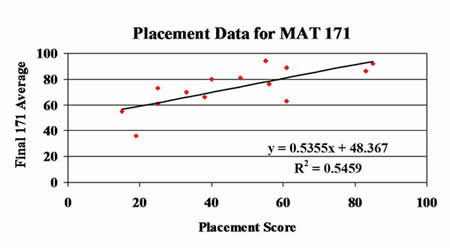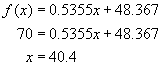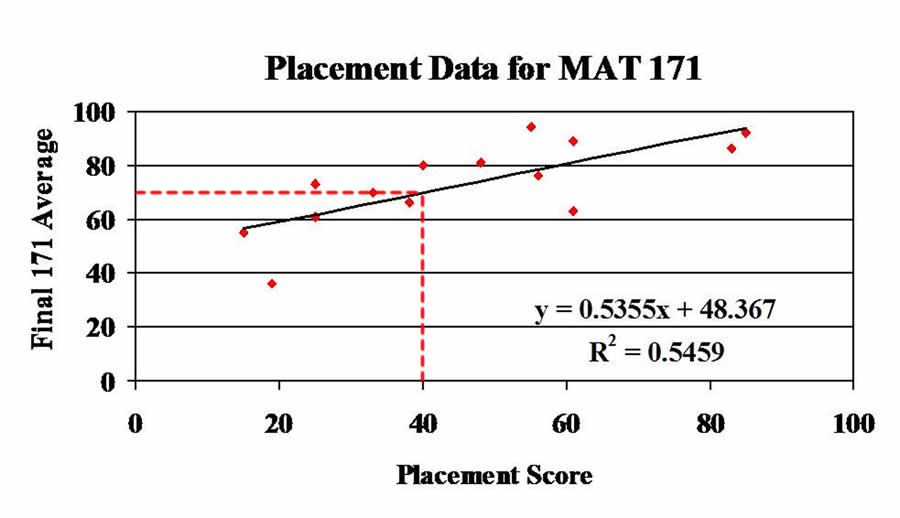# Placement Tests

## Here is a table of the data for a section of MAT 171. In the table x is the placement score and y is the final 171 average.

 x 61 40 19 25 61 48 38 56 33 55 85 25 15 83 y 63 80 36 61 89 81 66 76 70 94 92 73 55 86

## Here is a chart of the data along with the line of best fit.## Using the linear regression equation we can determine cut-off scores for the placement test. For instance, if we want to know what placement score will result in a final grade of C, we use 70 as final average in the regression equation.## So, a student making at least 40 on this placement test can expect to make at least a C in MAT 171.